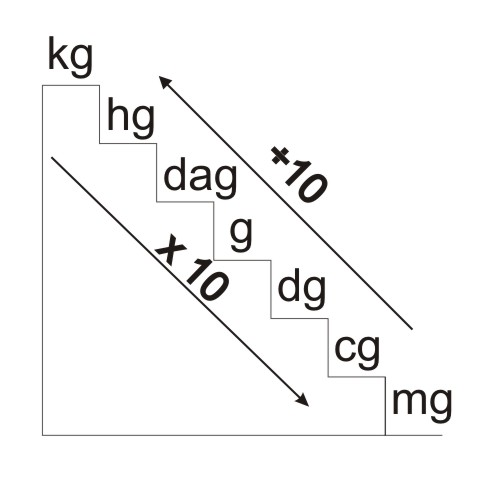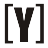# Kilograms [kg]

kilograms (kg) is a unit of mass / weight, kg is also the basic unit in the international unit system (SI). In the International Unit System, 1 kg = 1 liter of pure water with a temperature of 40° C. The tool to be used to determine the weight of an item is the scale.

The commonly used units of weight are kg, hg, dag, g, dg, cg, mg, ounces, pounds, quintal, and tons. To calculate weight units can use the conversion unit of the following units.• Each goes up one level divided by 10
• Each goes down one level at times with 10

Example: 1 kg how many grams?

How to convert: kg to g?

See the unit ladder above, from kg to gram (g) down 3 steps. So that it is obtained,

1 kg = 1 × 10 × 10 × 10 g

1 kg = 1 × 1000 g

1 kg = 1000 g

1 kg is 1000 g.

From the weight conversion unit stairs above can be obtained

1 kg = 1000 g
1 hg = 100 g
1 dag = 10 g
1 g = 1 g
1 dg = 0.1 g
1 cg = 0.01 g
1 mg = 0.001 g

Other Mass Units

1 ton = 1000 kg
1 quintal = 100 kg
1 ounce = 0.1 kg = 1 hg
1 pound = 0.5 kg = 5 ounces
1 carat = 0,0002 kg
1 cental = 45.35 kg
1 slug = 15.59 kg
1 stone = 6.35YoosFuhl.com Would you like to receive notifications on latest updates? No Yes